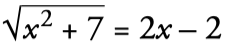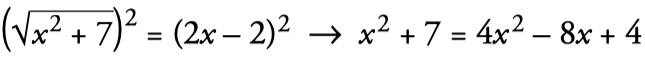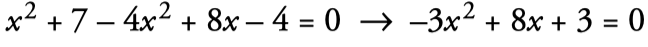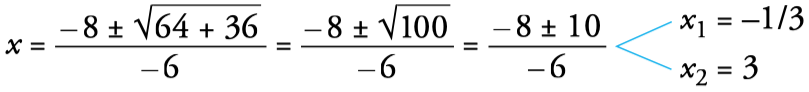Select Page

The quadratic equation is of the form ax2+bx+c=0 where a is nonzero. Depending on the values ​​that b and c have, we will be talking about complete equations or incomplete equations.

Complete quadratic equations are specific that have b and c other than zero and is solved by applying the following formula:

Example of a complete quadratic equation:

### Discriminant equation

The discriminant of the equation is used to know the number of solutions of an equation.

The incomplete quadratic equation is that in which b=0 or c=0 and can be solved very simply, without the need to apply any formula.

The factoring quadratics are those that have factor products in the form of paralysis in which an unknown variable appears. For its solution the factors are eliminated and it is solved with the formula of the complete equations.

Remember: to solve quadratic equations, that is, “product of several factors equaled to zero”, we equal each factor to zero and solve the corresponding equations.

Radical equations are those that contain roots. for example:

1. We isolate the radical in one member, passing the other to the other:2. We square the two members:3. We pass everything to a member and order it:4. We solve the obtained equation: (a=–3, b=8, c=3)5. In this type of radical equation, when squaring (2nd step), false solutions can appear. Therefore, it is necessary to check the solutions obtained by substituting them in the initial equation. In this case, x=–1/3 is not a solution, but x=3 is. The equation has a solution: x=3

1. Calculate the values ​​of the unknown and indicators from least to greatest:

## Ejercicios de ecuaciones de segundo grado

2. Calculate and indicate the smallest possible solutions:

## Ejercicios de ecuaciones de segundo grado

### Factors of 36

The number 36 has 9 factors and is a composite number. To calculate the factors of 36 we divide,...

### Factors of 35

The number 35 has 4 factors and is a composite number. To calculate the factors of 35 we divide,...

### Factors of 34

The number 4 has 4 factors and is a composite number. To calculate the factors of 34 we divide,...

### Factors of 33

The number 33 has 4 factors and is a composiite number. To calculate the factors of 33 we divide,...

### Factors of 32

The number 32 has 6 factors and is a composite number. To calculate the factors of 32 we divide,...

### Factors of 31

The number 31 has 2 factors and is a prime number. To calculate the factors of 31 we divide,...

### Factors of 30

The number 30 has 8 factors and is a composite number. To calculate the factors of 30 we divide,...

### Factors of 29

The number 29 has 2 factors and is a prime number. To calculate the factors of 29 we divide,...

### Factors of 28

The number 28 has 5 factors and is a composite number. To calculate the factors of 28 we divide,...

### Factors of 27

The number 27 has 4 factors and is a composite number. To calculate the factors of 27 we divide,...